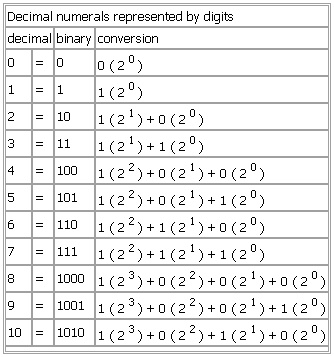# Decimal numerals represented by digits

Decimal numerals represented by digits

Table
Decimal numerals represented by digits
decimal binary conversion
0 = 0 0 ( 20 )
1 = 1 1 ( 20 )
2 = 10 1 ( 21 ) + 0 ( 20 )
3 = 11 1 ( 21 ) + 1 ( 20 )
4 = 100 1 ( 22 ) + 0 ( 21 ) + 0 ( 20 )
5 = 101 1 ( 22 ) + 0 ( 21 ) + 1 ( 20 )
6 = 110 1 ( 22 ) + 1 ( 21 ) + 0 ( 20 )
7 = 111 1 ( 22 ) + 1 ( 21 ) + 1 ( 20 )
8 = 1000 1 ( 23 ) + 0 ( 22 ) + 0 ( 21 ) + 0 ( 20 )
9 = 1001 1 ( 23 ) + 0 ( 22 ) + 0 ( 21 ) + 1 ( 20 )
10 = 1010 1 ( 23 ) + 0 ( 22 ) + 1 ( 21 ) + 0 ( 20 )
See as table:* * *

Universalium. 2010.

### Look at other dictionaries:

• Binary-coded decimal — In computing and electronic systems, binary coded decimal (BCD) is a digital encoding method for numbers using decimal notation, with each decimal digit represented by its own binary sequence. In BCD, a numeral is usually represented by four bits …   Wikipedia

• Arithmetic — tables for children, Lausanne, 1835 Arithmetic or arithmetics (from the Greek word ἀριθμός, arithmos “number”) is the oldest and most elementary branch of mathematics, used b …   Wikipedia

• Calculator — For mechanical precursors to the modern calculator, see mechanical calculator. For other uses, see Calculator (disambiguation). An electronic pocket calculator with a 7‑segment LCD display, that can perform basic arithmetic operations …   Wikipedia

• Calendar date — For the use of dates on Wikipedia, see the Manual of Style. A date in a calendar is a reference to a particular day represented within a calendar system. The calendar date allows the specific day to be identified. The number of days between two… …   Wikipedia

• Character encoding — Special characters redirects here. For the Wikipedia editor s handbook page, see Help:Special characters. A character encoding system consists of a code that pairs each character from a given repertoire with something else, such as a sequence of… …   Wikipedia